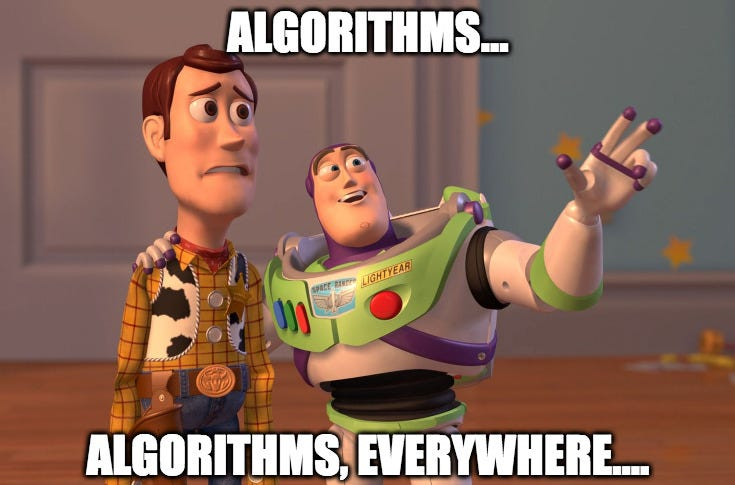# Once Again, about Greatest Common Divisor, the Euclidean Algorithm and a Little About the History of Algorithms in General. Of Course, with Swift Examples

Algorithms — one of the main topics of programming, they are everywhere (especially in job interviews, haha).How can one possibly do without an old joke in such a post?!

# The Euclidean Algorithm

Just out of curiosity, here is the Knuth’s edition of the Euclidian algorithm’s specification:

`func subtractionGCD(_ firstNumber: Int,                    _ secondNumber: Int) -> Int {    if let simpleGCD = simpleCasesGCD(firstNumber, secondNumber) {        return simpleGCD    }    var firstNumber = firstNumber    var secondNumber = secondNumber    while firstNumber != 0, secondNumber != 0 {        if firstNumber > secondNumber {            firstNumber = firstNumber - secondNumber        } else {            secondNumber = secondNumber - firstNumber        }    }    return firstNumber + secondNumber // One of them is 0.}`
`func simpleCasesGCD(_ firstNumber: Int,                    _ secondNumber: Int) -> Int? {    if firstNumber == secondNumber {        return firstNumber // Any.    }    if firstNumber == 0 {        return secondNumber    }    if secondNumber == 0 {        return firstNumber    }    return nil}`
`func divisionGCD(_ firstNumber: Int, _ secondNumber: Int) -> Int {    if let simpleGCD = simpleCasesGCD(firstNumber, secondNumber) {        return simpleGCD    }    var firstNumber = firstNumber    var secondNumber = secondNumber    while firstNumber != 0, secondNumber != 0 {        if firstNumber > secondNumber {            firstNumber = firstNumber % secondNumber        } else {            secondNumber = secondNumber % firstNumber        }    }    return firstNumber + secondNumber // One of them is 0.}`
`// Generates 100 pairs.let pairs = (0..<100).map { _ in    (Int.random(in: 0..<10000), Int.random(in: 0..<10000))}`
`func testSubstractionGCDPerformance() {    measure() {        _ = pairs.map { substractionGCD(\$0, \$1) }    }}`

## An “Improved” Version of the Euclidean Algorithm

It’s possible to came across the Euclidean algorithm’s version in which one of the numbers on every step is replaced, instead of a division remainder, by a difference between that remainder and the second number. This version can be implemented like this:

`func improvedDivisionGCD(_ firstNumber: Int,                         _ secondNumber: Int) -> Int {    if let simpleGCD = simpleCasesGCD(firstNumber, secondNumber) {        return simpleGCD    }    var firstNumber = firstNumber    var secondNumber = secondNumber    while firstNumber != 0, secondNumber != 0 {        if firstNumber > secondNumber {            let firstNumberClaim = firstNumber % secondNumber            if firstNumberClaim > secondNumber / 2 {                firstNumber = abs(firstNumberClaim - secondNumber)            } else {                firstNumber = firstNumberClaim            }        } else {            let secondNumberClaim = secondNumber % firstNumber            if secondNumberClaim > firstNumber / 2 {                secondNumber = abs(secondNumberClaim - firstNumber)            } else {                secondNumber = secondNumberClaim            }        }    }    return firstNumber + secondNumber // One of them is 0.}`

## A Little Bit More about Significance of the Euclidean Algorithm

The algorithm also has the geometric version, for the greatest measure of two line segments finding.

## Complexity

The algorithm’s time complexity was researched quite some time ago, not too fast and by considerably more learned people than your humble servant. However, the problem is solved and the question is answered. By Gabriel Lamé.

# Binary Algorithm

Talking about GCD search it’s worth mention another algorithm that was proposed in the 1960s by someone called Joseph Stein. The algorithm is focused on binary arithmetics and doesn’t use division operations.

1. If u is even and v is not, then gcd(u, v) = gcd(u ÷ 2, v);
2. gcd(u, v) = gcd(u — v, v) (follows from the Euclidean algorithm);
3. If both u and v are odd, then (u — v) is even and |u — v| < max(u, v).
`func binaryGCD(_ firstNumber: Int, _ secondNumber: Int) -> Int {    if let simpleGCD = simpleCasesGCD(firstNumber, secondNumber) {        return simpleGCD    }    var firstNumber = firstNumber    var secondNumber = secondNumber    var shift = 0    while (firstNumber | secondNumber) & 1 == 0 {        shift += 1        firstNumber >>= 1        secondNumber >>= 1    }    while firstNumber & 1 == 0 {        firstNumber >>= 1    }    repeat {        while secondNumber & 1 == 0 {            secondNumber >>= 1        }        if firstNumber > secondNumber {            swap(&firstNumber, &secondNumber)        }        secondNumber -= firstNumber    } while secondNumber != 0    return firstNumber << shift}`

# Conclusion

To be frank, I didn’t want to prove anything or draw any revolutionary conclusions from this simple “research”. I just wanted to satisfy my curiosity, to check different approaches results and to warm up my fingers. However, I hope it was interesting to you, too!

Swift and general programming topics, Agile software development, soft skills

## More from Nikita Lazarev-Zubov

Swift and general programming topics, Agile software development, soft skills